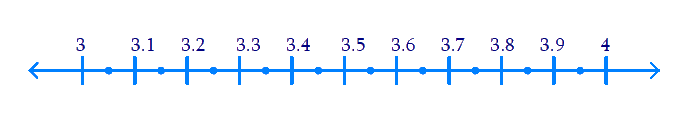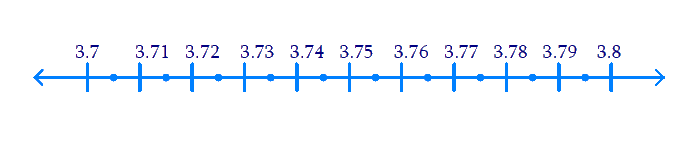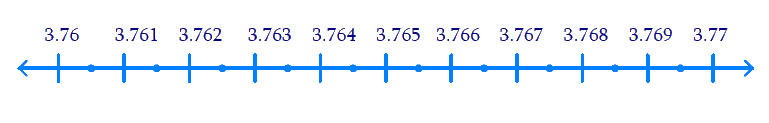# Ex.1.4 Q1 Number System Solution - NCERT Maths Class 9

Go back to  'Ex.1.4'

## Question

Visualize \begin{align} 3.765\end{align} on the number line, using successive magnification.

Video Solution
Number Systems
Ex 1.4 | Question 1

## Text Solution

Steps:

(i) $$3.7$$ lies between $$3$$ & $$4$$. So we divide the portion between $$3$$ & $$4$$. on the number line into $$10$$ equal parts$$3.76$$ lies between $$3.7$$ and $$3.8$$. So, we divide the portion between $$3.7$$ and $$3.8$$ on the number line into $$10$$ equal parts.$$3.765$$ lies between $$3.76$$ and $$3.77$$ and $$3.77$$ .Dividing the line before we gotVideo Solution
Number Systems
Ex 1.4 | Question 1

Learn from the best math teachers and top your exams

• Live one on one classroom and doubt clearing
• Practice worksheets in and after class for conceptual clarity
• Personalized curriculum to keep up with school